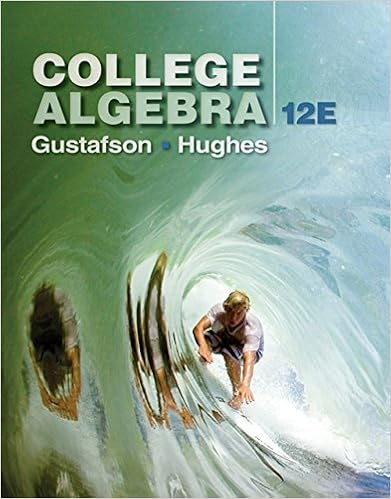# Identify the b half it square it add it to both sides

• 19

This preview shows page 13 - 19 out of 19 pages.

##### We have textbook solutions for you!
The document you are viewing contains questions related to this textbook.The document you are viewing contains questions related to this textbook.
Chapter 1 / Exercise 78
College Algebra
Gustafson/HughesExpert Verified
3. Identify the b# (half it - square it - add it to both sides)(Since there are no #’s to half—literally showmultiplying by 21)Square this “expression part”—add it to both sides4. Write Expression as a perfect square trinomialSimplify the other side (adding fractions need common denominators)--Answer should be in Standard Form.5. Square root both sidesPut a on the right in front of term(Fractions already have Common Denominator) – justwrite final expression.6. NOW you have a new formula called theQuadratic Formula(you can remember this formula through a song)To remember formula sing/hum the phase below to the “pop goes the weasel song”“x =’s negative b, plus or minus the square root of b2minus 4 a c, all over 2 a”******WRITE THE FORMULA DOWN AS YOU SING THE SONG******Steps for using the Quadratic Formula: (Use when quad equation= 0)Get equation equal to ZERO!!!Put Equation in standard form: __________________________Identify the a, b, and c #’s.Plug into the formula and simplify.
##### We have textbook solutions for you!
The document you are viewing contains questions related to this textbook.The document you are viewing contains questions related to this textbook.
Chapter 1 / Exercise 78
College Algebra
Gustafson/HughesExpert Verified
Solve the following using the quadratic formula, answers should be in simplest radical form when possible.1)Solve for x: x2– 12x = -20 2)Solve for x: 2x2+ 9x = 18Quadratic Formula:3) Solve for x: x2– 5x – 3 = 04) Solve for x:2x2– 4x = 114
•••